# Difference between revisions of "0708-1300/Class notes for Thursday, October 11"

Announcements go here

## Today's Agenda: Intro to Sard

• The Cantor set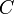$C$.
• Sets of measure zero.
•$C$ is of measure zero.
• But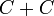$C+C$ is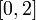$[0,2]$ (in two ways).
• Thick Cantor sets.
• "Measure zero" is a smooth invariant.
• Measure 0 makes sense on manifolds.
• Baby Sard for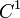$C^1$ functions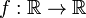$f:{\mathbb R}\to{\mathbb R}$:
• Such an$f$ with a Cantor set of singular points and values.
• And yet a proof.
• A counterexample for Sard for$C^1$ functions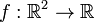$f:{\mathbb R}^2\to{\mathbb R}$.

## The Cantor Aerogel

Strange as it may seem, the faded and barely visible Cantor aerogel in the square at the bottom right of the image above is still thick enough to block all diagonal light rays.

Proof:

Take an arbitrary diagonal line intersecting the figure. Observe that in the first step figure the condition holds (the diagonal line intersects the black squares). Observe now that when moving to the second figure the given line will intersect a black square too. This means that if a diagonal line intersects the black set then it will intersect the set of the next step.

Since in each step the set is self similar i.e. in each quarter of the square you have the black set of the previous step, then the intersection point of the diagonal line is contained in the same quarter of the previous intersection. Since this quarters are nested and the diagonal of the quarters tents to zero, the sequence of intersection form a convergent sequence which converges inside the square (which is compact) and inside the segment of the line inside the square (which is compact too). Since the Cantor set is closed the limit point is in the Cantor set. It is inside the line segment as well.

QED

## Class Notes

Note: Assignment 1 was handed back

Definition 1a The Cantor Set.

Consider the closed interval [0,1] and denote it by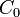$C_0$. Remove the open middle third leaving two closed intervals. Denote these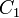$C_1$. Iteratively, remove the open middle third of any remaining closed interval to form a sequence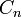$C_n$ each containing a disjoint union of closed intervals.

We define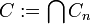$C:=\bigcap C_n$

Alternate Definition 1b

Consider a number in [0,1] written in "trinary", that is as a "decimal" expansion only in base three instead. Hence a number can be represented by say 0.01211... The cantor set simply removes all possible such sequences with 1's in them which correspond to the "middle" of a given segment. Note there is a slight ambiguity here for the same reason that 0.3999999... = 4 in the normal decimal expansion.

Claim

Since the number of possible sequences of 0's and 2's is uncountable, clearly C is uncountable.

Defintion 2

A set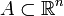$A\subset\mathbb{R}^n$ is of measure zero if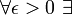$\forall \epsilon>0\ \exists$ a countable number of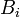$B_i$ such that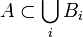$A\subset\bigcup_{i}B_i$ and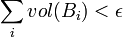$\sum_{i}vol(B_i)<\epsilon$

Claim 1

C is of measure zero

Proof$C_n$, and hence C, can be covered by a union of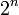$2^n$ intervals of length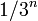$1/3^n$ and hence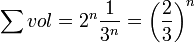$\sum vol = 2^n \frac{1}{3^n} = \left(\frac{2}{3}\right)^n$ which converges to zero as n tends to infinity.

Claim 2

If$A\subset\mathbb{R}^n$ and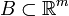$B\subset\mathbb{R}^m$ are of measure zero then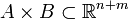$A\times B\subset\mathbb{R}^{n+m}$ is of measure zero.

Proof

Let A be coverd by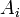$A_i$, and B by$B_i$ (which,respectively, have volumes that sum less than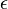$\epsilon$ )

But then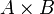$A\times B$ is covered by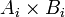$A_i\times B_i$ which has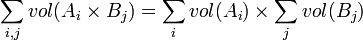$\sum_{i,j} vol(A_i\times B_j)= \sum_{i}vol(A_i)\times\sum_{j}vol(B_{j})$ which can be made arbitrarily small by assumption.

Claim 3

A countable union of measure zero sets is measure zero

Proof

Let each$A_i$ in the countable union be covered by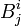$B_{j}^{i}$ so that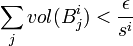$\sum_{j}vol(B_{j}^{i})<\frac{\epsilon}{s^i}$

Hence,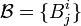$\mathcal{B} = \{B_{j}^{i}\}$ covers the union and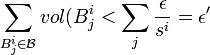$\sum_{B^{i}_{j}\in\mathcal{B}}vol(B^{i}_{j}<\sum_{j}\frac{\epsilon}{s^i} = \epsilon'$

Q.E.D

Claim 4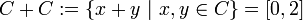$C+C:=\{x+y\ |\ x,y\in C\} = [0,2]$

Comment: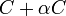$C+\alpha C$ is not necessarily going to be a full interval; indeed, it could have measure zero depending on the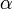$\alpha$

Proof 1

C+C takes points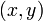$(x,y)$ in the Cantor Aerogel (demonstrated pictorially above) into the real line by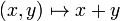$(x,y)\mapsto x+y$

I.e., this is a diagonal projection by adding the components.

Clearly this is true for the first iteration. If it is true for the first iteration, one can apply the same reasoning to the second iteration which just consists of blocks that are precisely the first iteration, and so on. A compactness argument (done above) then shows this is true not just for any C_n but indeed for the intersection C.

Proof 2

This is a highly pictorial argument, but loosely one looks at the diagram from a 45 degree angle and at the first iteration sees a horizontal line. At the second iteration one sees a horizontal line with the middle third duplicated vertically above and below its original location. And so on iteratively. It is thus clear from the picture that there is never going to be a possible vertical line that won't hit something.

Proof 3

Let C =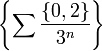$\left\{ \sum\frac{\{0,2\}}{3^n}\right\}$

So C/2 =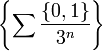$\left\{ \sum\frac{\{0,1\}}{3^n}\right\}$

And so C/2 + C/2 =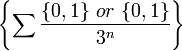$\left\{ \sum\frac{\{0,1\}\ or\ \{0,1\}}{3^n}\right\}$ =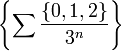$\left\{ \sum\frac{\{0,1,2\}}{3^n}\right\}$ = [0,1]

Just multiply by 2 to get the result.

Q.E.D

Comment

As in proof 1, we have the idea that if$C+\alpha C$ lets light through in the first instance, it will continue doing so in subsequent instances,perhaps even letting increasingly more light through until it lets all the light through except for a set of measure zero.

Notation / Definition 3

We call the C defined above the Cantor set and we call any set that is homeomorphic to C a Cantor set.

Example 1 / Defintion 4

Consider the construction which takes the unit interval, removes the middle 10th. Then removes the middle 100th from the resulting intervals, then removes the middle 100th, etc. This construction is clearly homeomorphic to that of the original C. However, this set does NOT have measure zero and hence we call it a thick Cantor Set.

Comment: The previous example shows that a set having measure zero is not a topological property, as we might expect given that a measure is designed to correspond with our intuitive notion of "volume" which is clearly not relevant for arbitrary topological spaces.

However,

Claim 5

If A has measure 0 in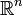$\mathbb{R}^n$ and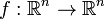$f:\mathbb{R}^n\rightarrow\mathbb{R}^n$ is$C^1$ then f(A) is measure zero in$\mathbb{R}^n$.

Comment

Note this is NOT true for a domain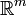$\mathbb{R}^m$ where m>n. As a quick example of this consider a line in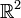$\mathbb{R}^2$ and the canonical projection to a line in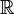$\mathbb{R}$. The line in$\mathbb{R}^2$ has trivial measure as it can be covered by n boxes with sides 1/n and hence volume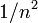$1/n^2$. Thus the volume of this covering is 1/n which can be made arbitrarily small. However, the line in$\mathbb{R}$ has non trivial measure.

Proof of Claim 5

W.L.O.G.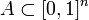$A\subset[0,1]^n$. In [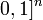$0,1]^n$ df is bounded, say, by M. Hence, a set of diameter$\epsilon$ will get mapped by f to a set of diameter at most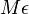$M\epsilon$ (This is essentially Mean Value Theorem)

Hence, a cube of sides$\epsilon$ maps to a cube of size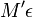$M'\epsilon$ where the prime just takes into account the fixed difference between the length of a side and that of a diagonal.

Hence, let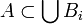$A\subset\bigcup B_i$. Then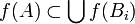$f(A)\subset\bigcup f(B_i)$ which has volume less then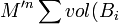$M'^n\sum vol (B_i$) which we can make arbitrarily small.

Q.E.D

Claim 6

1) Sard's Theorem is true for merely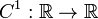$C^1:\mathbb{R}\rightarrow\mathbb{R}$. However,

2) It is FALSE for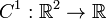$C^1:\mathbb{R}^2\rightarrow\mathbb{R}$

As such a counterexample we consider a function f whose critical points are the C but whose critical values are a cantor set. Such a function has been given explicitly here.

We then construct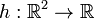$h:\mathbb{R}^2\rightarrow\mathbb{R}$ by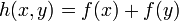$h(x,y) = f(x) + f(y)$ which has critical points that are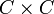$C\times C$ and critical values are f(C)+f(C).